In :
#@title Licensed under the Apache License, Version 2.0 (the "License");
# you may not use this file except in compliance with the License.
# You may obtain a copy of the License at
#
#
# Unless required by applicable law or agreed to in writing, software
# WITHOUT WARRANTIES OR CONDITIONS OF ANY KIND, either express or implied.
# See the License for the specific language governing permissions and

In :
#@title MIT License
#
# Copyright (c) 2017 François Chollet
#
# Permission is hereby granted, free of charge, to any person obtaining a
# copy of this software and associated documentation files (the "Software"),
# to deal in the Software without restriction, including without limitation
# the rights to use, copy, modify, merge, publish, distribute, sublicense,
# and/or sell copies of the Software, and to permit persons to whom the
# Software is furnished to do so, subject to the following conditions:
#
# The above copyright notice and this permission notice shall be included in
# all copies or substantial portions of the Software.
#
# THE SOFTWARE IS PROVIDED "AS IS", WITHOUT WARRANTY OF ANY KIND, EXPRESS OR
# IMPLIED, INCLUDING BUT NOT LIMITED TO THE WARRANTIES OF MERCHANTABILITY,
# FITNESS FOR A PARTICULAR PURPOSE AND NONINFRINGEMENT. IN NO EVENT SHALL
# THE AUTHORS OR COPYRIGHT HOLDERS BE LIABLE FOR ANY CLAIM, DAMAGES OR OTHER
# LIABILITY, WHETHER IN AN ACTION OF CONTRACT, TORT OR OTHERWISE, ARISING
# FROM, OUT OF OR IN CONNECTION WITH THE SOFTWARE OR THE USE OR OTHER
# DEALINGS IN THE SOFTWARE.


# Basic regression: Predict fuel efficiency¶

In a regression problem, we aim to predict the output of a continuous value, like a price or a probability. Contrast this with a classification problem, where we aim to select a class from a list of classes (for example, where a picture contains an apple or an orange, recognizing which fruit is in the picture).

This notebook uses the classic Auto MPG Dataset and builds a model to predict the fuel efficiency of late-1970s and early 1980s automobiles. To do this, we'll provide the model with a description of many automobiles from that time period. This description includes attributes like: cylinders, displacement, horsepower, and weight.

This example uses the tf.keras API, see this guide for details.

In :
# Use seaborn for pairplot
!pip install -q seaborn

In :
from __future__ import absolute_import, division, print_function, unicode_literals

import pathlib

import matplotlib.pyplot as plt
import pandas as pd
import seaborn as sns

try:
# %tensorflow_version only exists in Colab.
%tensorflow_version 2.x
except Exception:
pass
import tensorflow as tf

from tensorflow import keras
from tensorflow.keras import layers

print(tf.__version__)

1.14.0


## The Auto MPG dataset¶

The dataset is available from the UCI Machine Learning Repository.

### Get the data¶

In :
dataset_path = keras.utils.get_file("auto-mpg.data", "http://archive.ics.uci.edu/ml/machine-learning-databases/auto-mpg/auto-mpg.data")
dataset_path

Out:
'C:\\Users\\muzay\\.keras\\datasets\\auto-mpg.data'

Import it using pandas

In :
column_names = ['MPG','Cylinders','Displacement','Horsepower','Weight',
'Acceleration', 'Model Year', 'Origin']
na_values = "?", comment='\t',
sep=" ", skipinitialspace=True)

dataset = raw_dataset.copy()
dataset.tail()

Out:
MPG Cylinders Displacement Horsepower Weight Acceleration Model Year Origin
393 27.0 4 140.0 86.0 2790.0 15.6 82 1
394 44.0 4 97.0 52.0 2130.0 24.6 82 2
395 32.0 4 135.0 84.0 2295.0 11.6 82 1
396 28.0 4 120.0 79.0 2625.0 18.6 82 1
397 31.0 4 119.0 82.0 2720.0 19.4 82 1

### Clean the data¶

The dataset contains a few unknown values.

In :
dataset.isna().sum()

Out:
MPG             0
Cylinders       0
Displacement    0
Horsepower      6
Weight          0
Acceleration    0
Model Year      0
Origin          0
dtype: int64

To keep this initial tutorial simple drop those rows.

In :
dataset = dataset.dropna()


The "Origin" column is really categorical, not numeric. So convert that to a one-hot:

In :
origin = dataset.pop('Origin')

In :
dataset['USA'] = (origin == 1)*1.0
dataset['Europe'] = (origin == 2)*1.0
dataset['Japan'] = (origin == 3)*1.0
dataset.tail()

Out:
MPG Cylinders Displacement Horsepower Weight Acceleration Model Year USA Europe Japan
393 27.0 4 140.0 86.0 2790.0 15.6 82 1.0 0.0 0.0
394 44.0 4 97.0 52.0 2130.0 24.6 82 0.0 1.0 0.0
395 32.0 4 135.0 84.0 2295.0 11.6 82 1.0 0.0 0.0
396 28.0 4 120.0 79.0 2625.0 18.6 82 1.0 0.0 0.0
397 31.0 4 119.0 82.0 2720.0 19.4 82 1.0 0.0 0.0

### Split the data into train and test¶

Now split the dataset into a training set and a test set.

We will use the test set in the final evaluation of our model.

In :
train_dataset = dataset.sample(frac=0.8,random_state=0)
test_dataset = dataset.drop(train_dataset.index)


### Inspect the data¶

Have a quick look at the joint distribution of a few pairs of columns from the training set.

In :
sns.pairplot(train_dataset[["MPG", "Cylinders", "Displacement", "Weight"]], diag_kind="kde")

Out:
<seaborn.axisgrid.PairGrid at 0x2326a0f6c08>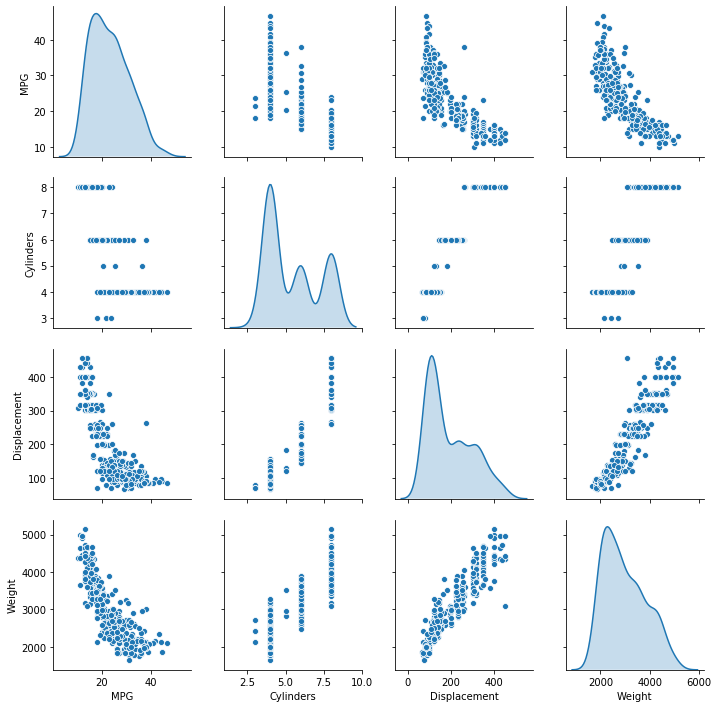Also look at the overall statistics:

In :
train_stats = train_dataset.describe()
train_stats.pop("MPG")
train_stats = train_stats.transpose()
train_stats

Out:
count mean std min 25% 50% 75% max
Cylinders 314.0 5.477707 1.699788 3.0 4.00 4.0 8.00 8.0
Displacement 314.0 195.318471 104.331589 68.0 105.50 151.0 265.75 455.0
Horsepower 314.0 104.869427 38.096214 46.0 76.25 94.5 128.00 225.0
Weight 314.0 2990.251592 843.898596 1649.0 2256.50 2822.5 3608.00 5140.0
Acceleration 314.0 15.559236 2.789230 8.0 13.80 15.5 17.20 24.8
Model Year 314.0 75.898089 3.675642 70.0 73.00 76.0 79.00 82.0
USA 314.0 0.624204 0.485101 0.0 0.00 1.0 1.00 1.0
Europe 314.0 0.178344 0.383413 0.0 0.00 0.0 0.00 1.0
Japan 314.0 0.197452 0.398712 0.0 0.00 0.0 0.00 1.0

### Split features from labels¶

Separate the target value, or "label", from the features. This label is the value that you will train the model to predict.

In :
train_labels = train_dataset.pop('MPG')
test_labels = test_dataset.pop('MPG')


### Normalize the data¶

Look again at the train_stats block above and note how different the ranges of each feature are.

It is good practice to normalize features that use different scales and ranges. Although the model might converge without feature normalization, it makes training more difficult, and it makes the resulting model dependent on the choice of units used in the input.

Note: Although we intentionally generate these statistics from only the training dataset, these statistics will also be used to normalize the test dataset. We need to do that to project the test dataset into the same distribution that the model has been trained on.

In :
def norm(x):
return (x - train_stats['mean']) / train_stats['std']
normed_train_data = norm(train_dataset)
normed_test_data = norm(test_dataset)


This normalized data is what we will use to train the model.

Caution: The statistics used to normalize the inputs here (mean and standard deviation) need to be applied to any other data that is fed to the model, along with the one-hot encoding that we did earlier. That includes the test set as well as live data when the model is used in production.

## The model¶

### Build the model¶

Let's build our model. Here, we'll use a Sequential model with two densely connected hidden layers, and an output layer that returns a single, continuous value. The model building steps are wrapped in a function, build_model, since we'll create a second model, later on.

In :
def build_model():
model = keras.Sequential([
layers.Dense(64, activation='relu', input_shape=[len(train_dataset.keys())]),
layers.Dense(64, activation='relu'),
layers.Dense(1)
])

optimizer = tf.keras.optimizers.RMSprop(0.001)

model.compile(loss='mse',
optimizer=optimizer,
metrics=['mae', 'mse'])
return model

In :
model = build_model()


### Inspect the model¶

Use the .summary method to print a simple description of the model

In :
model.summary()

Model: "sequential_2"
_________________________________________________________________
Layer (type)                 Output Shape              Param #
=================================================================
dense_6 (Dense)              (None, 64)                640
_________________________________________________________________
dense_7 (Dense)              (None, 64)                4160
_________________________________________________________________
dense_8 (Dense)              (None, 1)                 65
=================================================================
Total params: 4,865
Trainable params: 4,865
Non-trainable params: 0
_________________________________________________________________


Now try out the model. Take a batch of 10 examples from the training data and call model.predict on it.

In :
example_batch = normed_train_data[:10]
example_result = model.predict(example_batch)
example_result

Out:
array([[ 1.9389942e-02],
[ 4.2831272e-02],
[ 6.1084151e-01],
[ 4.4380873e-04],
[-1.4150590e-01],
[ 1.8100436e-01],
[-1.2327269e-01],
[-2.1228668e-01],
[ 2.0383289e-01],
[-1.4790146e-01]], dtype=float32)

It seems to be working, and it produces a result of the expected shape and type.

### Train the model¶

Train the model for 1000 epochs, and record the training and validation accuracy in the history object.

In :
# Display training progress by printing a single dot for each completed epoch
class PrintDot(keras.callbacks.Callback):
def on_epoch_end(self, epoch, logs):
if epoch % 100 == 0: print('')
print('.', end='')

EPOCHS = 1000

history = model.fit(
normed_train_data, train_labels,
epochs=EPOCHS, validation_split = 0.2, verbose=0,
callbacks=[PrintDot()])

....................................................................................................
....................................................................................................
....................................................................................................
....................................................................................................
....................................................................................................
....................................................................................................
....................................................................................................
....................................................................................................
....................................................................................................
....................................................................................................

Visualize the model's training progress using the stats stored in the history object.

In :
hist = pd.DataFrame(history.history)
hist['epoch'] = history.epoch
hist.tail()

Out:
loss mean_absolute_error mean_squared_error val_loss val_mean_absolute_error val_mean_squared_error epoch
995 2.543425 1.009911 2.543425 9.247956 2.273298 9.247956 995
996 2.470314 0.985320 2.470313 9.226539 2.264675 9.226540 996
997 2.590764 0.985387 2.590764 9.621528 2.300281 9.621528 997
998 2.532930 0.987139 2.532930 9.620787 2.292989 9.620787 998
999 2.669562 1.022631 2.669563 9.539299 2.299247 9.539299 999
In :
def plot_history(history):
hist = pd.DataFrame(history.history)
hist['epoch'] = history.epoch

In :
hist.head()

Out:
loss mean_absolute_error mean_squared_error val_loss val_mean_absolute_error val_mean_squared_error epoch
0 561.569505 22.363611 561.569458 550.538101 22.080034 550.538086 0
1 508.788988 21.172859 508.788971 494.725562 20.798456 494.725555 1
2 456.599142 19.916399 456.599091 435.657018 19.337883 435.657013 2
3 400.991760 18.504139 400.991730 373.148433 17.671095 373.148438 3
4 343.021601 16.898037 343.021576 308.461039 15.806126 308.461060 4
In :
  plt.figure()
plt.xlabel('Epoch')
plt.ylabel('Mean Abs Error [MPG]')
plt.plot(hist['epoch'], hist['mean_absolute_error'],
label='Train Error')
plt.plot(hist['epoch'], hist['val_mean_absolute_error'],
label = 'Val Error')
plt.ylim([0,5])
plt.legend()

plt.figure()
plt.xlabel('Epoch')
plt.ylabel('Mean Square Error [$MPG^2$]')
plt.plot(hist['epoch'], hist['mean_squared_error'],
label='Train Error')
plt.plot(hist['epoch'], hist['val_mean_squared_error'],
label = 'Val Error')
plt.ylim([0,20])
plt.legend()
plt.show()

plot_history(history)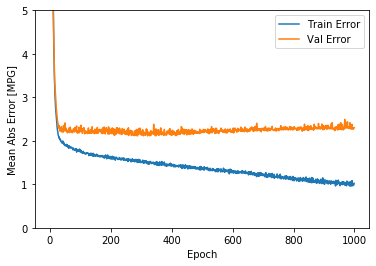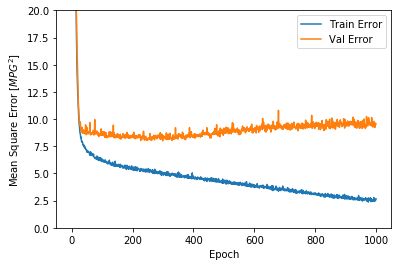This graph shows little improvement, or even degradation in the validation error after about 100 epochs. Let's update the model.fit call to automatically stop training when the validation score doesn't improve. We'll use an EarlyStopping callback that tests a training condition for every epoch. If a set amount of epochs elapses without showing improvement, then automatically stop the training.

In :
model = build_model()

# The patience parameter is the amount of epochs to check for improvement
early_stop = keras.callbacks.EarlyStopping(monitor='val_loss', patience=10)

history = model.fit(normed_train_data, train_labels, epochs=EPOCHS,
validation_split = 0.2, verbose=0, callbacks=[early_stop, PrintDot()])

plot_history(history)

..............................................................

The graph shows that on the validation set, the average error is usually around +/- 2 MPG. Is this good? We'll leave that decision up to you.

Let's see how well the model generalizes by using the test set, which we did not use when training the model. This tells us how well we can expect the model to predict when we use it in the real world.

In :
loss, mae, mse = model.evaluate(normed_test_data, test_labels, verbose=2)

print("Testing set Mean Abs Error: {:5.2f} MPG".format(mae))

78/78 - 0s - loss: 7.0945 - mean_absolute_error: 2.1272 - mean_squared_error: 7.0945
Testing set Mean Abs Error:  2.13 MPG


### Make predictions¶

Finally, predict MPG values using data in the testing set:

In :
test_predictions = model.predict(normed_test_data).flatten()

plt.scatter(test_labels, test_predictions)
plt.xlabel('True Values [MPG]')
plt.ylabel('Predictions [MPG]')
plt.axis('equal')
plt.axis('square')
plt.xlim([0,plt.xlim()])
plt.ylim([0,plt.ylim()])
_ = plt.plot([-100, 100], [-100, 100])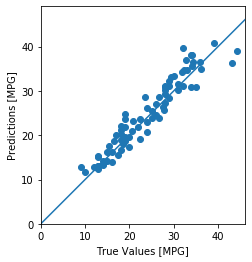It looks like our model predicts reasonably well. Let's take a look at the error distribution.

In :
error = test_predictions - test_labels
plt.hist(error, bins = 25)
plt.xlabel("Prediction Error [MPG]")
_ = plt.ylabel("Count")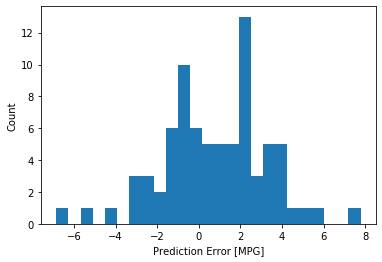It's not quite gaussian, but we might expect that because the number of samples is very small.

## Conclusion¶

This notebook introduced a few techniques to handle a regression problem.

• Mean Squared Error (MSE) is a common loss function used for regression problems (different loss functions are used for classification problems).
• Similarly, evaluation metrics used for regression differ from classification. A common regression metric is Mean Absolute Error (MAE).
• When numeric input data features have values with different ranges, each feature should be scaled independently to the same range.
• If there is not much training data, one technique is to prefer a small network with few hidden layers to avoid overfitting.
• Early stopping is a useful technique to prevent overfitting.